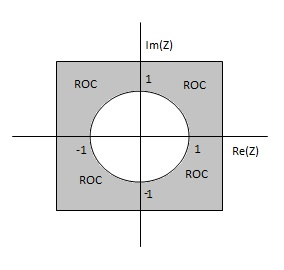0

## Z Transform of U(n)

• Find the Z Transform and ROC of U(n) ?

The Z transform of a discrete time signal x(n) is given by,

Here given x(n) = u(n)

Therefore,

We know that U(n) = 1; n ≥ 0
= 0; n < 0
Therefore,

X(z) = Z0 + Z-1 +Z-2 + Z-3 + Z-4 +………….
= 1 + Z-1 +Z-2 + Z-3 + Z-4 +………….

It is clear that the infinite series is a Geometric Progression (GP)

The sum of the GP is given by

Sum = First Term / (1 – Common Ratio)

The common ratio (r) is given by

r = second term/first term
= third term/second term

So, r = Z-1 /1 = Z-2/ Z-1 = Z-1

Hence, the sum of the series is given by

### ROC of U(n)

The ROC of U(n) is given by

|r| < 1

|Z-1| < 1

|1/Z| < 1

|Z| > |1|ROC of U(n)